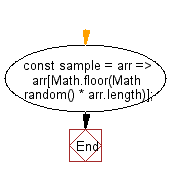# JavaScript: Get a random element from an array

## JavaScript fundamental (ES6 Syntax): Exercise-149 with Solution

Write a JavaScript program to get a random element from an array.

• Use Math.random() to generate a random number.
• Multiply it by Array.prototype.length and round it off to the nearest whole number using Math.floor().
• This method also works with strings.

Sample Solution:

JavaScript Code:

``````//#Source https://bit.ly/2neWfJ2
const sample = arr => arr[Math.floor(Math.random() * arr.length)];
console.log(sample([3, 7, 9, 11]));
```
```

Sample Output:

```11
```

Pictorial Presentation:Flowchart:Live Demo:

See the Pen javascript-basic-exercise-149-1 by w3resource (@w3resource) on CodePen.

Improve this sample solution and post your code through Disqus

What is the difficulty level of this exercise?

Test your Programming skills with w3resource's quiz.

﻿